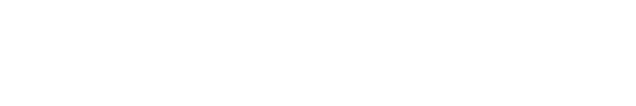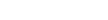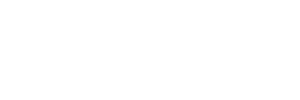```# 二值化图片
@staticmethod
def two_value_img(img_path, threshold):
img = Image.open(img_path).convert('L')
#  setup a converting table with constant threshold
tables = []
for i in range(256):
if i``````# 根据图片特点，自己写的降噪算法
def clean_img(img, threshold):
width, height = img.size
for j in range(height):
for i in range(width):
point = img.getpixel((i, j))
if point == 0:
for x in range(threshold):
if j + x >= height:
break
else:
if point != img.getpixel((i, j + x)):
img.putpixel((i, j), 1)
break
return img

# 八值法降噪
def clean_img_eight(img, threshold):
width, height = img.size
arr = [[0 for col in range(width)] for row in range(height)]
arr = array(arr)
for j in range(height):
for i in range(width):
point = img.getpixel((i, j))
if point == 0:
sum = 0
for x in range(-1, 2):
for y in range(-1, 2):
if i + x > width - 1 or j + y > height - 1 or \
i + x < 0 or j + y < 0:
sum += 1
else:
sum += img.getpixel((i + x, j + y))
if sum >= threshold:
arr[j, i] = 1

for i in range(len(arr)):
for j in range(len(arr[i])):
if arr[i, j] == 1:
img.putpixel((j, i), 1)
return img```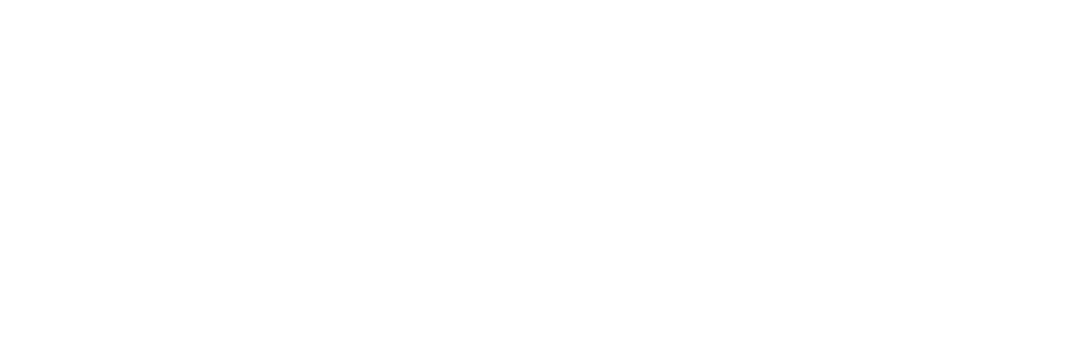```# CFS图像切割
@staticmethod
def cut_img(img, threshold, cut_width, cut_height, width_min, width_max, height_min):
charters_imgs = []
width, height = img.size
charters_pixels = []
visited_pixels = []
pixel_arr = ImgTools.get_pixel_arr(img)
for i in range(width):
for j in range(height):
pixel = img.getpixel((i, j))
if pixel == 0 and [i, j] not in visited_pixels:
charter_pixels = Node(i, j, pixel_arr, []).traversal()
visited_pixels.extend(charter_pixels)
if len(charter_pixels) > threshold:
charters_pixels.append(charter_pixels)
for i in range(len(charters_pixels)):
x_min = 0
y_min = 0
x_max = 0
y_max = 0
# 这里是为了处理没有粘连但是垂直方向有重合的字符
width, height = img.size
tmp_img = Image.new('1', (width * 2, height * 2), 255)
for j in range(len(charters_pixels[i])):
x, y = charters_pixels[i][j]
tmp_img.putpixel((x, y), 0)
if x > x_max:
x_max = x
else:
if x < x_min or x_min == 0:
x_min = x
if y > y_max:
y_max = y
else:
if y < y_min or y_min == 0:
y_min = y
if width_min < x_max - x_min < width_max and y_max - y_min > height_min:
# charters_imgs.append(tmp_img.crop((x_min, y_min, x_max, y_max)))
# 这里是为了将所有的图片切成一样大，便于后期的特征提取
charters_imgs.append(tmp_img.crop((x_min, y_min, x_min + cut_width, y_min + cut_height)))
return charters_imgs```

```class Node:
x = 0
y = 0
graph_arr = []
visited_neighbors = []

def __init__(self, x, y, graph_arr, visited_neighbors):
self.x = x
self.y = y
self.graph_arr = graph_arr
self.visited_neighbors = visited_neighbors

def traversal(self):
for i in range(-1, 2):
for j in range(-1, 2):
p = self.x + i
q = self.y + j
if (0```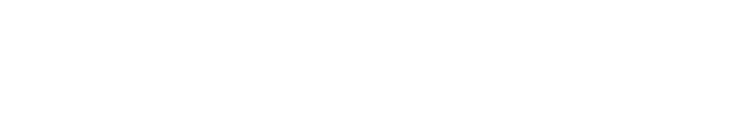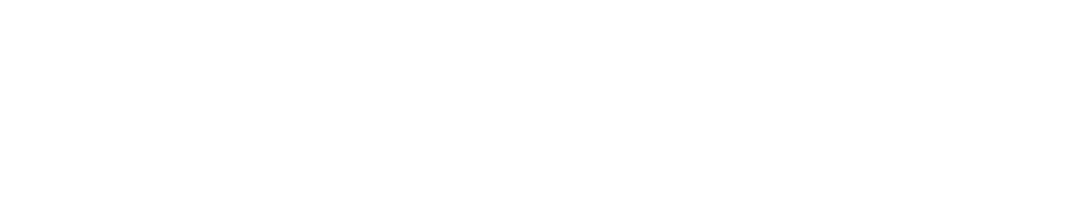✎   训练模型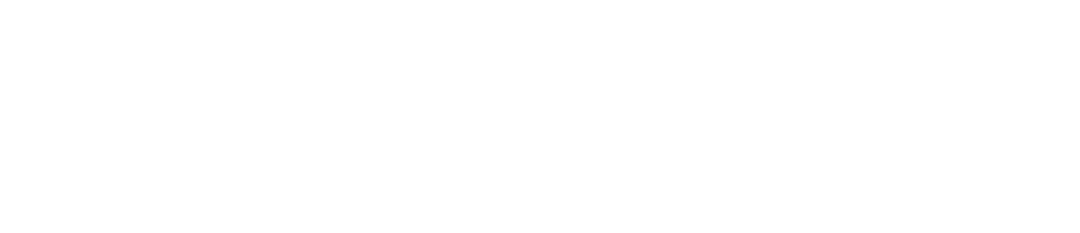1、目前整个识别流程已经走通，验证码识别服务也初具对外服务的能力；

2、虽然目前对于整体验证码的识别效果不是很好，但是，验证码服务拼的是识别率，比如说一个验证码需要识别，我在对其进行预处理和切割之后发现字符粘连效果不好，则完全可以抛弃，这并不影响识别率。换句话讲我只是别切出来是四个字符的验证码即可（如果遇到一个网站每个图片的粘连都比较严重，这条路就走不通了）；

3、优化方向有两个：

（1）优化切割字符的算法，目前的机器学习算法在图片切割比较好的情况下识别率是非常高的，因此目前这类验证码的切割是整个过程中的难点，对于该案例可以采用波斯平滑后通过垂直投影图找到极值点作为水滴法的起点是一个思路；

（2）增加样本量，目前是40个识别率已经可以接受，如果增加训练集的Size识别率应该会有所提升。

## 基于神经网络的图片验证码识别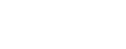## 识别过程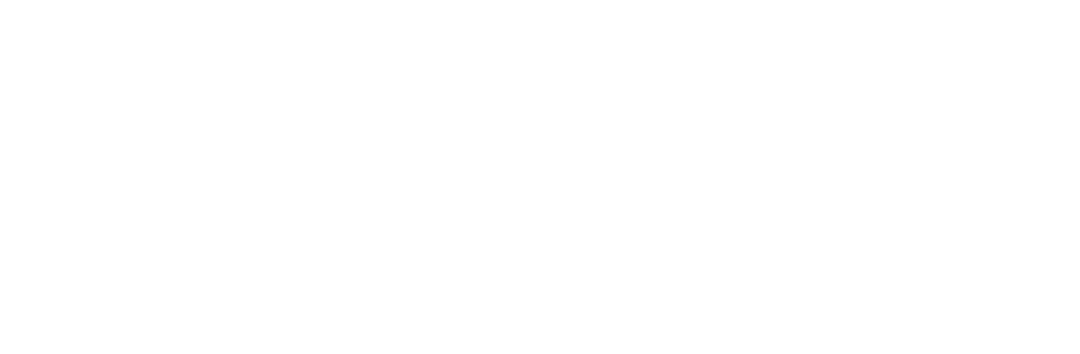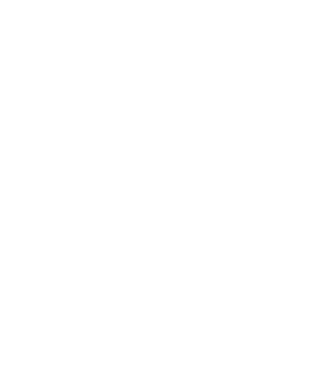CNN:训练集样本量为3500时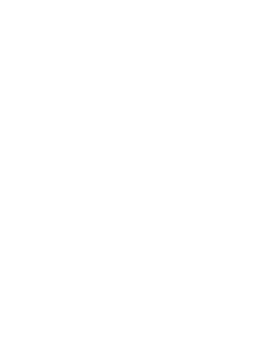CNN:训练集样本量为4500时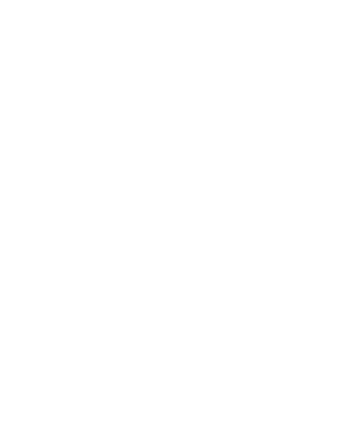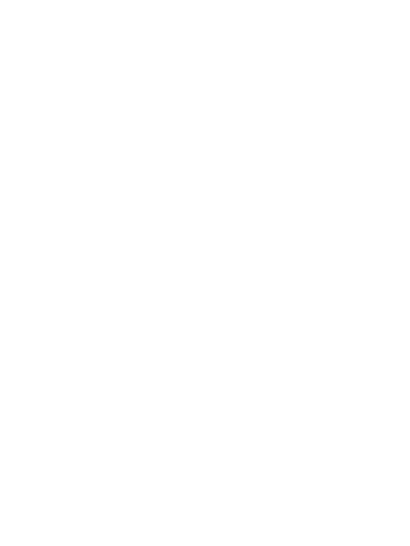be a reasonable crawler